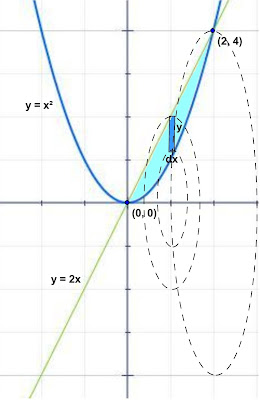## Tuesday, April 9, 2013

### Volume - Solid Revolution, 2

Category: Integral Calculus, Analytic Geometry

"Published in Newark, California, USA"

Find the volume generated by revolving about the x-axis and y-axis of the area bounded by the following curves

Solution:

To illustrate the problem, it is better to sketch the graph of the two equations above using the principles of Analytic Geometry as followsPhoto by Math Principles in Everyday Life

From the figure above, there are two points of intersection between the two curves. Solve the systems of two equations two unknowns in order to get the coordinates of the points of intersection as follows

Equate

and

we have,

Equate each factor to zero and solve for the value of x as follows

If
then

If

then

Therefore, their points of intersection are (0, 0) and (2, 4).

Next, from the given two equations above, it is better to use a vertical strip at the area bounded by two curves and label further the figure as followsPhoto by Math Principles in Everyday Life

If you rotate the shaded area about the x-axis, the vertical strip becomes a ring as followsPhoto by Math Principles in Everyday Life

The volume of a ring formed by the rotation of a vertical strip about the x-axis is

Integrate on both sides of the equation to get the volume of a solid formed by the rotation of the area about the x-axis as follows

If you rotate the shaded area about the y-axis, the vertical strip becomes a cylindrical shell as followsPhoto by Math Principles in Everyday Life

The volume of a cylindrical shell formed by the rotation of a vertical strip about the y-axis is

The circumference of the base of the cylindrical shell is 2πx. x is the distance of the vertical strip to the axis of revolution which is the y-axis. y is the height of a cylindrical shell. dx is the thickness of a cylindrical shell. Since dx is a very small value, then the two radii of the cylindrical shell are almost the same which is x. The three dimensions of a thin rectangular box are 2πx, y, and dx. When you wrapped a thin rectangular box into a cylinder, then it becomes a cylindrical shell. Therefore, the volume of a cylindrical shell is

Integrate on both sides of the equation to get the volume of a solid formed by the rotation of the area about the y-axis as follows